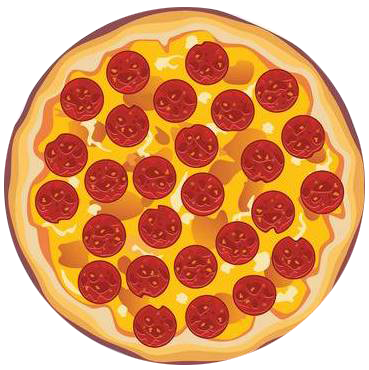IntroductionIntroductionWe've always found that the easiest way to understand adding and subtracting fractions is by seeing 👀 fractions in action.

Pick whichever you vibe with more:

INTRO
Think of a fraction like this:The
denominator
,
, tells us the size of the slice. The
numerator
,
, tells us how many slices we have of that size.
CALCULATOR

KEY STEPS

## How to Add & Subtract Fractions

### Step 4. Add/subtract the numerators, and keep the denominator the same.

LESSON
Multiplying by the Denominators

## Multiplying by the Denominators

A reliable way to find the common denominator is to multiply the two denominators together.

But, when we do this, we have to make sure we multiply both the top and bottom by the same thing. Look what happens if we only multiply the denominators:

 OriginalMultiply by Denominator NewY’all see what we see?! 👀 What happened to all our pizza?! When we only multiply the denominators, the amount of pizza changes, and we end up solving an entirely different problem.
PRACTICE
Multiplying by the Denominators

## Practice: Multiplying Denominators

Question 1 of 3:

### Step 1. Check the denominators to see if they're the same.Are the denominators the same?

LESSON
Using the LCM

## Using the LCM

We can also find a common denominator by finding the LCM, or least common multiple, of the two denominators.

### Is Using the LCM Better than Multiplying by the Denominators? 🤔

Tbh… it depends on what comes more naturally to you. 🤷🏻
Both methods will always get you to the correct answer, but you may end up having to deal with larger numbers when multiplying by the denominators.
In addition, using the LCM can come in pretty handy when solving problems that involve more than two fractions.
Let's try an example!
PRACTICE
Using the LCM

## Practice: Using the LCM

Question 1 of 3:

### Step 1. Check the denominators to see if they're the same.Are the denominators the same?

CONCLUSION
Sheesh, look at you go! Thanks for checking out this lesson ☺️🙏.Leave Feedback